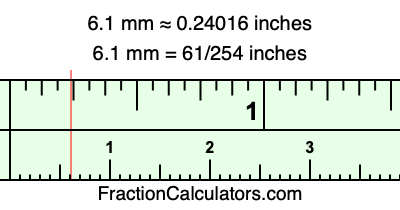6.1 mm in inchesHere is how to convert 6.1 mm to inches. We will give you the fractional answer, the decimal answer, and illustrate the answer on our tape measure.

One millimeter is equal to 5/127 inches, and 6.1 as a fraction is 61/10. Therefore, to get the fractional answer to 6.1 mm in inches, we multiply 61/10 by 5/127 and simplify if necessary.

cm × 5/127 = inches
61/10 × 5/127 = inches
61/10 × 5/127 = 61/254
61/10 mm = 61/254 inches
6.1 mm = 61/254 inches

As promised above, we also have the answer to 6.1 mm in inches in decimal form. Although the fractional answer above is exact, the decimal answer may be rounded if necessary:

61/254 ≈ 0.240157480314961
61/254 ≈ 0.24016
6.1 mm ≈ 0.24016 inches

Our image below shows 6.1 mm on a tape measure. The top row of the tape measure is inches, and each mark on the bottom row is 1 millimeter (10 millimeter marks equal 1 centimeter).As you can see, we drew a red line where 6.1 mm and 0.24016 inches meet on the measuring tape.

Millimeters to Inches Calculator
Here you can convert another length in millimeters to inches.

Convert  mm to inches.

What is 6.2 mm in inches?
Here is another inches to millimeters calculation that we have done for you.